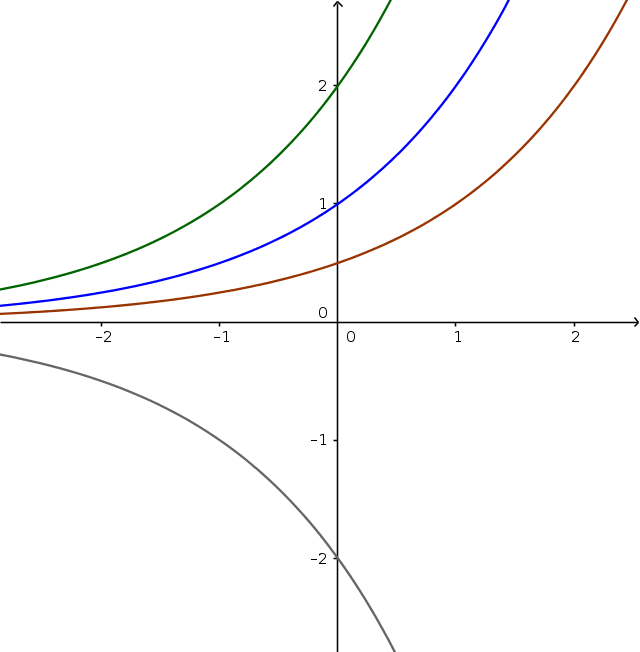Math Exponential functions Stretching and compressing

Stretching and compressing

The graph of an exponential function is stretched or compressed with the factor $a$ parallel to the y-axis. The general formula is:

$y=a\cdot b^x$
!

Remember

• If $a > 1$, the graph is stretched.
• If $0 < a < 1$, the graph is compressed.

• If $a < 0$, the graph is reflected
$\color{blue}{f(x)=2^x}$
$\color{green}{g(x)=2\cdot2^x}$ (stretched)
$\color{brown}{h(x)=\frac12\cdot2^x}$ (compressed)
$\color{grey}{i(x)=-2\cdot2^x}$ (reflected)